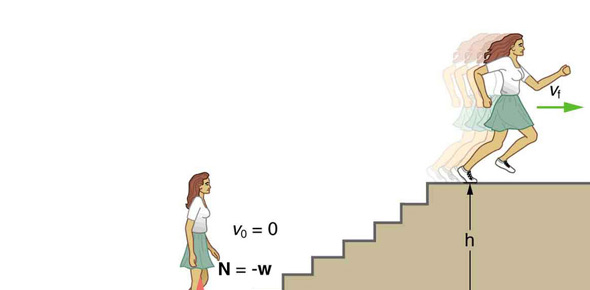# Physics Module #8: Work And Energy

7 Questions | Attempts: 221
ShareSettings• 1.
Energy is...
• A.

The product of the displacement of an object and the component of the applied force that is parallel to the displacement

• B.

The product of the work of and object and the displacement

• C.

The ability to do work

• D.

The ability to belch loudly

• 2.
Work is...
• A.

The product of the displacement of an object and the component of the applied force that is parallel to the displacement

• B.

The product of the work of and object and the displacement

• C.

The ability to use energy

• D.

The ability to double knot a ribbon

• 3.
Which work equation is accurate?
• A.

Work=parallel force times the displacement

• B.

Work=mass times gravity times the displacement

• 4.
A rolling ball is an example of potential energy.
• A.

True

• B.

False

• 5.
A crashing plane is an example of kinetic energy.
• A.

True

• B.

False

• 6.
Choose the correct equation.
• A.

PE=(1/2)mv squared

• B.

PE=mgh

• C.

KE=mg

• D.

KE=mgh

• 7.
Choose the correct equation.
• A.

KE=(1/2)mgh

• B.

PE=(1/2)mgh

• C.

KE=(1/2)mv squared

• D.

PE=(1/2)mv squared

## Related TopicsBack to top
×

Wait!
Here's an interesting quiz for you.